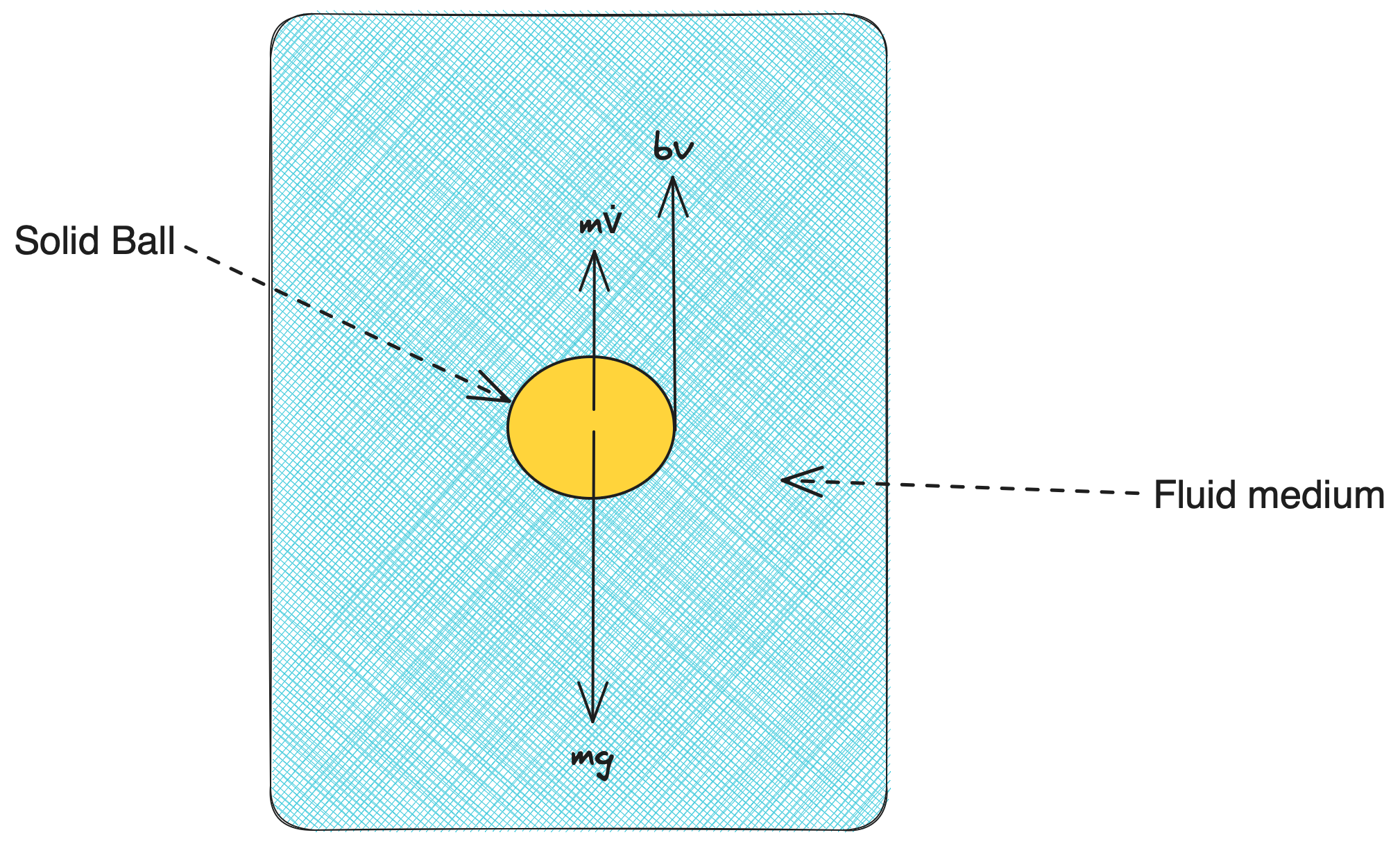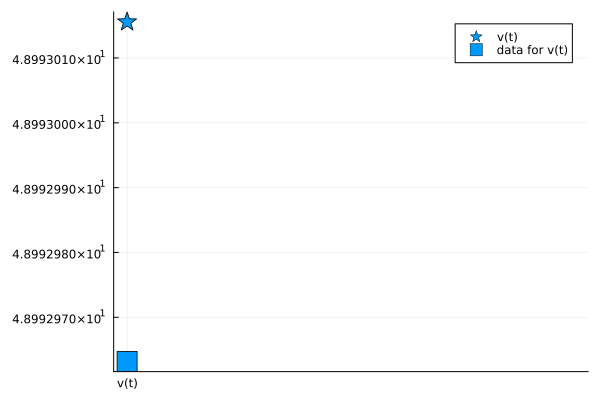# Terminal Velocity attained by a Ball in a Viscous Medium

In this example, we will demonstrate the scope of simulating SteadyStateExperiment types, using JuliaSimModelOptimizer. Here, we will consider a ball encountering a drag-force in a viscous medium. From the classical Stoke's Law, assuming a small spherical object falling in a medium, we can describe a linear relationship between drag-force and velocity, where k is defined as a constant, ${6 \pi \eta r v}$, as below:

\begin{aligned} F_v &= k*v \\ \end{aligned}

## Julia Environment

using JuliaSimModelOptimizer
using JuliaSimModelOptimizer: get_params
using ModelingToolkit
using OrdinaryDiffEq
using DataFrames
using StatsPlots
using OptimizationOptimJL
using DataSets
using CSV
using Plots

## Model setup

In this case, we have considered the effect of two governing forces, the viscous-drag force and the self-weight of the ball, while the ball is travelling through the fluid. Following the Newton's 2nd Law of motion in the vertical direction, we can generate the following equation from the force-balance equation:

\begin{aligned} \frac{d(v)}{dt} &= g - (b/m)*v \\ \end{aligned}Here, we have two parameters, namely g as the acceleration due to gravity, and k as the constant b/m which takes into account the effect of the viscous drag-force.

iv = only(@variables t)
states = @variables v(t)=0
D = Differential(t)
ps = @parameters g=9.8 k=0.2
eqs = [D(v) ~ g - k*v]
model = ODESystem(eqs, iv, states, ps, name = :terminalvelocity)
\begin{align} \frac{\mathrm{d} v\left( t \right)}{\mathrm{d}t} =& g - k v\left( t \right) \end{align}

## Generating Data with Normally Distributed Noise added

In order to generate the data, we have simulated the SteadyStateProblem using the built-in SteadyStateSolver DyanmicSS. As our actual data will inherently gather some noise due to measurement error etc, in order to reflect the practical scenarios, we have in-corporated a Gaussian random noise in our data.

function generate_noisy_data(model;
params = [],
u0 = [],
noise_std = 0.1)
sol = solve(prob, DynamicSS(Rodas4()))

df = sol
rd = randn(1)*noise_std
df.u .+= rd
return df
end

data = generate_noisy_data(model)
u: 1-element Vector{Float64}:
48.992963190407714

Assuming the ball reaches steady-state in Inf secs (which is to signify that it reaches steady-state terminal velocity after a long period of time), we can now create the data-frame with this timestamp information.

ss_data = DataFrame("timestamp" => Inf, "v(t)" => data.u)
1×2 DataFrame
Rowtimestampv(t)
Float64Float64
1Inf48.993

## Creating Inverse Problem from Steady State Experiment

Once we have generated the data when the ball attains its steady state, we need to create the SteadyStateExperiment for calibration.

experiment = SteadyStateExperiment(ss_data, model; alg = DynamicSS(Rodas4()))
invprob = InverseProblem(experiment, model, [k => (0, 1)])
InverseProblem for terminalvelocity with one experiment optimizing 1 parameters.


## Calibrating the model

r = calibrate(invprob, SingleShooting(maxiters = 10^3))
Calibration result computed in 32 seconds. Final objective value: 2.74161e-9.
Optimization ended with Success Return Code and returned.

┌──────────┐
│        k │
├──────────┤
│ 0.200028 │
└──────────┘


We can see that the optimizer has converged to a sufficiently small loss value. Let us now validate the prediction with respect to the actual parameter used in the data-generation. We can see that the recovered parameter k matches well with its true value, 0.2.

## Visualizing the optimized parameters

plot(experiment, invprob, r, show_data = true, legend = true)We can ascertain that our model performs well as the relative difference between the predicted terminal-velocity and the actual data is reasonably small (~ 1e-5).